Courses

# Test: Functions

## 15 Questions MCQ Test Mathematics (Maths) Class 11 | Test: Functions

Description
This mock test of Test: Functions for JEE helps you for every JEE entrance exam. This contains 15 Multiple Choice Questions for JEE Test: Functions (mcq) to study with solutions a complete question bank. The solved questions answers in this Test: Functions quiz give you a good mix of easy questions and tough questions. JEE students definitely take this Test: Functions exercise for a better result in the exam. You can find other Test: Functions extra questions, long questions & short questions for JEE on EduRev as well by searching above.
QUESTION: 1

Solution:
QUESTION: 2

### If X = {1, 2, 3, 4}, Y = {1, 2, 3,…., 20}, and f: X → Y be the correspondence which assigns each element in X the value equal to its square, then the domain, co-domain and range of f is

Solution:

The correct option is B

Since the function is defined from

XY.So the domain will be the set X which is {1,2,3,4}. Codomain is  what may possibly come out of a function. So codomain is the whole set Y which is {1,2,3,...,20}. Range is The range is the set of possible output values which will be the values in the set of Y which have a pre-image. That is, the value of squares. So the range is {1,4,,9,16}.

QUESTION: 3

### Which of the following is not true?

Solution:

A relation f from a set A to a set B is said to be function if every element of set A has one and only one image in set B. In other words, a function f is a relation such that no two pairs in the relation has the same first element. The notation f : X →Y means that f is a function from X to Y.

QUESTION: 4

The domain of the function f(x)=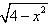is all real x such that

Solution:

4 - x2 = 0
=> (2+x)(2-x) = 0
x = +-2
Now select a test point, let it be x = 0. Then y = (4 - (0)2)1/2 = 2, so the function is defined on [-2,2]
Thus, the graph of y = (4 - x2)1/2 is a semicircle with radius 2 and domain is [-2,2]

QUESTION: 5

Let X = {1, 2, 3, 4}, Y = {1, 2, …, 10} and f : X –> Y be defined by f(x) = 2x + 1, x ∈ X. Then the range of f is

Solution:

X = {1, 2, 3, 4}
Y = {1, 2, …, 10}
f: X→ Y be defined by
f(x) = 2x + 1
f (1) = 3
f (2) = 5
f (3) = 7
f (4) = 9
Range(f) = {3, 5, 7, 9}

QUESTION: 6

If f(x) = x2 – x + 1; g(x) = 7x – 3, be two real functions then (f + g)(3) is

Solution:

f(x) = x2 – x + 1; g(x) = 7x – 3
(f+g)(x) = (x2 - x + 1 + 7x - 3)
=(x2 - x + 7x + 1 - 3)
= x2 + 6x - 2
(f+g)(3) = x2 + 6x - 2
= (3)2 + 6(3) - 2
= 9 + 18 - 2
= 25

QUESTION: 7

Which of the following relations is not a function?

Solution:

A function is a relation in which no two ordered pairs have the same first element. A function associates each element in its domain with one and only one element in its range. R={(1,2), (1,4)(3,1),(5,1)} is not  a function because all the first elements are not different.

QUESTION: 8

The following arrow diagram of the function f : A → A be defined as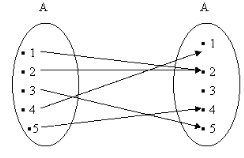Solution:
QUESTION: 9

If A = {1,2,3}, B = {a,b,c}, which of the following is a function?

Solution:

Option A has value b & c for 2.
Option C has value b & c for 1.
Option D has value a & c for 1.

QUESTION: 10

Which of the relations is not a function?

Solution:

Example: y = x2
If x = 2, y = 4.
If x = -2, y = 4. So there you have a many-to-one relation and it is DEFINITELY a function.
Now, a one-to-many relation is NOT a function.

QUESTION: 11

The domain and range of the function f: R → R defined by: f = {(x+1, x+5): x ∈ {0, 1, 2, 3, 4, 5}}

Solution:
QUESTION: 12

Identify the domain and range of the function f(x), defined in N, as below: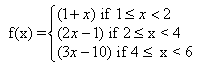Solution: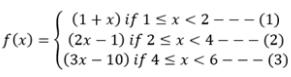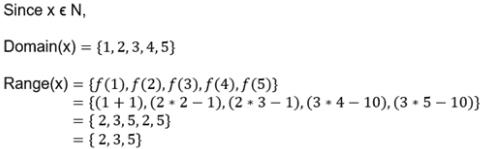QUESTION: 13

If A = {2, 4, 6, 8} and B = {2, 3, 5, 7, 9}. Which of the following is a function from A to B?

Solution:
QUESTION: 14

Find the domain of the function f(x) = x |x|

Solution: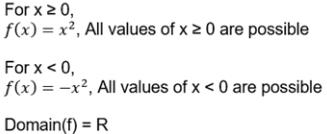QUESTION: 15

Find the domain of the function f(x) = 3x/2x - 8

Solution:

f(x) = 3x/2x-8
denominator can't be zero so
2x - 8 not equal to 0
x should not equal to 4
we have domain R - {4}